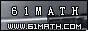Today is . WELCOME: huohai3983/44663  Home | Reg Login | All user | setHomePage | BookmarkHome | Math Test Online | Addition | Subtraction | Multiplication | Division | Time | Worksheet(JPG/PDF) | CAI | Flash Games |Recommendation articleArticle searchHome--maths--First Grade--Subtraction Equations - One Digit Numbers

Subtraction Equations - One Digit Numbers

Subtraction equations - 1 digit

An equation is a mathematical statement such that the expression on the left side of the equals sign (=) has the same value as the expression on the right side. An example of an equation is 6 - 4 = 2.

One of the terms in an equation may not be know and needs to be determined. Often this unknown term is represented by a letter such as x. (e.g. x - 4 = 2).

The solution of an equation is finding the value of the unknown x. To find the value of x we can use the additive equation property which says: The two sides of an equation remain equal if the same number is added to each side.

Example:
x - 5 = 7
x - 5 + 5 = 7 + 5
x - 0 = 12
x = 12
Check the answer by substituting the value of x (12) back into the equation.
12 - 5 = 7

#### What number would complete the sentence?

 -     =

2006-12-14 22:40:05
Viewed 1706 times CloseGreatmathsites

### Translate

 Thank you to online math channels' Mathematics Department for hosting these lessons. Copyright © 2006 - 2008 GZtrain Inc. All Rights Reserved